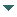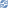# gvSIG

##### Personal tools
You are here: Home Register operations
gvSIG DesktopgvSIG Desktop

Cached time 11/21/13 11:24:39# Register operations

Up one level
 ◄ Previous Create operations Next: Operation invocation ►

This document explains how to register a new operation associated to a new geometry

If we want to use an operation, it must be registered previously. If we try to execute an operation and it isn't registered by the GeometryManager, we will get an exception.

The operation registration must be done in a class extended from the GeometryLibrary, in the postinitialize method. The registerGeometryOperation method takes care of the registration of an operation. The operations must be registered indicating the concrete geometry type (or several geometry types) which are associated with them. For that, can be operations that only are valid in a subset of geometries.

For example, the PrintLn operation commented in the previous section can be executed by all geometry types. To do it, is necessary to register it following this steps:

```GeometryManager geometryManager = GeometryLocator.getGeometryManager();
geometryManager.registerOperation("PrintLn", printLn);
```

The registerOperation method accepts two parameters: the first one is the name of the operation and the second one is the object which implements the operation. In this case, we are registering the draw operation in the stardard output and it's associated to all geometries.

There's a mechanism to register operations associated with a concrete geometry types. We can create, for example, an operation PrintLn to write only 2-dimensional points. The operation will be the next:

```public class printLnPoint2D extends GeometryOperation {
public static final String NAME = "println";
public static final int CODE = GeometryLocator.getGeometryManager().getGeometryOperationCode(NAME);

public int getOperationIndex() {
return CODE;
}

public Object invoke(Geometry geom, GeometryOperationContext ctx) throws GeometryOperationException {
Point point = (Point)geom;
System.out.println("Point 2D, X=" + point.getX() + " Y=" + point.getY());
return null;
}
}
```

This operation only works with objects of the type 2-dimensional point. For that, in the method registerOperation we must specify two new parameters: the type and subtype of the geometry which can use the operation. When the PrintLn operation is invoked, the code of the operation PrintLn will be executed for all the geometry types except to the 2-dimensional Point, which will execute the specific operation.

```GeometryManager geometryManager = GeometryLocator.getGeometryManager();
geometryManager.registerOperation("PrintLn", printLnPoint2D, Geometry.TYPES.Point, Geometry.SUBTYPES.GEOM2D);
```

The registerOperation method is overwritted to let us to associate an operation to all the geometry of a concrete type (for all the subtypes). This example shows how to do an operation that prints points, independently of their dimensions.

```public class printLnPoint extends GeometryOperation {
public static final String NAME = "println";
public static final int CODE = GeometryLocator.getGeometryManager().getGeometryOperationCode(NAME);

public int getOperationIndex() {
return CODE;
}

public Object invoke(Geometry geom, GeometryOperationContext ctx) throws GeometryOperationException {
Point point = (Point)geom;
System.out.println("Point, ");
for (int i=0 ; i<point.getDimension() ; i++){
System.out.println("Coordinate i =" + point.getCoordinateAt(i));
}
return null;
}
}
```

To register this operation, we will execute this steps:

```GeometryManager geometryManager = GeometryLocator.getGeometryManager();
geometryManager.registerOperation("PrintLn", printLnPoint, Geometry.TYPES.Point);
```

The last option is to register an operation for all the geometries of the same subtype. A clearly example of this operation is the 2-dimensional draw, which can be executed for all the 2-dimensional geometries. To register this operation we must execute:

```geometryManager.registerOperationBySubtype("Draw2D", draw2D, Geometry.SUBTYPES.GEOM2D);
```
 ◄ Previous Create operations Next: Operation invocation ►